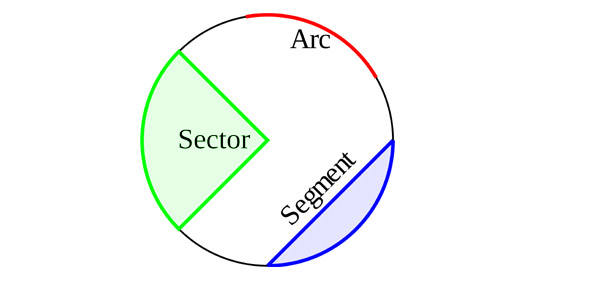# Parts Of A Circle

Approved & Edited by ProProfs Editorial Team
The editorial team at ProProfs Quizzes consists of a select group of subject experts, trivia writers, and quiz masters who have authored over 10,000 quizzes taken by more than 100 million users. This team includes our in-house seasoned quiz moderators and subject matter experts. Our editorial experts, spread across the world, are rigorously trained using our comprehensive guidelines to ensure that you receive the highest quality quizzes.
| By Dleichtman
D
Dleichtman
Community Contributor
Quizzes Created: 1 | Total Attempts: 445
Questions: 5 | Attempts: 447SettingsFor other shapes such as squares and rectangles it is easy to believe that it has parts. When it comes to a circle, it becomes hard to name the parts. The quiz below gauges your understanding on the subject.

• 1.

### Where is the circumfrences?

• A.

In The Middle.

• B.

The Edge of the circle

• C.

The Circles edge.

• D.

Or B and C.

D. Or B and C.
Explanation
The correct answer is "Or B and C" because the question is asking about the location of the circumferences, which are the boundaries or edges of a circle. Option B states "The Edge of the circle" and option C states "The Circles edge," both of which correctly describe the location of the circumferences. Therefore, the answer is that the circumferences can be found at the edge of the circle, as stated in options B and C.

Rate this question:

• 2.

### Where is The center?

• A.

In the center of the circle.

• B.

The edge of the circle.

• C.

A line starting in the middle and goes to the edge.

A. In the center of the circle.
Explanation
The correct answer is "in the center of the circle" because the center of a circle is the point that is equidistant from all points on the circumference. It is the point from which all radii of the circle are equal in length. Therefore, the center is located at the middle of the circle, regardless of its size or dimensions.

Rate this question:

• 3.

curcumfrenes
• 4.

• 5.

### Is the center part of the circle?

• A.

Yes

• B.

No

• C.

I Don`t Know

A. Yes
Explanation
The answer is yes because the center part of a circle is always located at the exact midpoint of the circle. It is the point from which all points on the circumference of the circle are equidistant. Therefore, by definition, the center is an integral part of the circle.

Rate this question:

Related TopicsBack to top